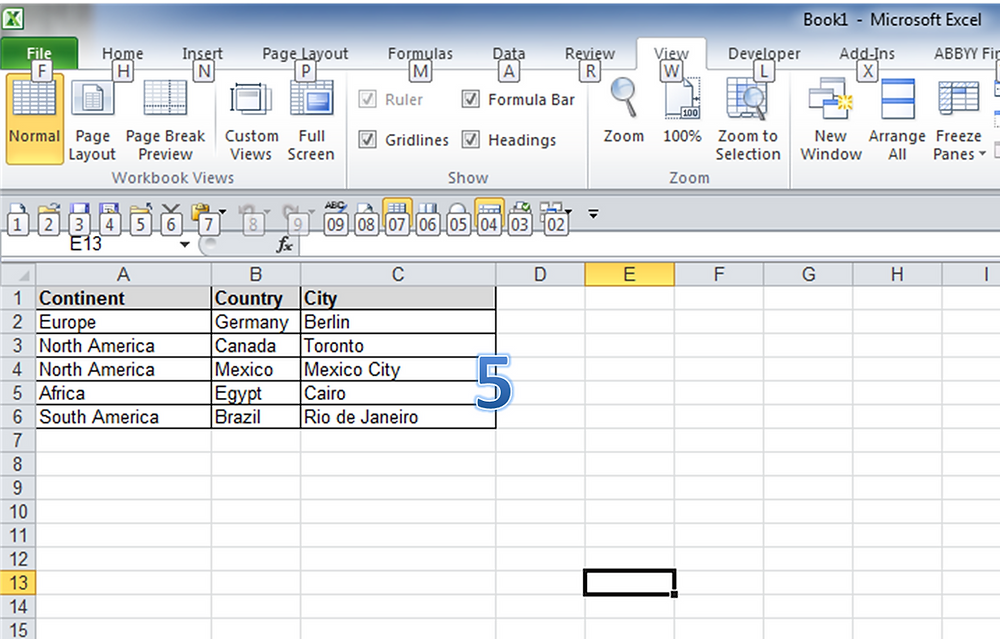Search

# IF. . . THEN Delete - Excel macro to automatically delete rows

Tonight I found a macro online which will automatically delete rows in Excel when the entry in one column equals a value that you specify. The code is available at this site:

Follow these steps when you want to delete rows with a particular value in column A. In this example shown in the screen grabs at the end of the post, I am taking out the entries for all of the cities located in Asia.

1. Press ALT + F11 and right click on VBAProject and choose Insert . . . Module.

2. Then paste in the code shown below.

3. On the lines which read:

If .Value = "ron" Then .EntireRow.Delete

'This will delete each row with the Value "ron"

. . . enter the values you need to specify in column A.

Save and close Visual Basic.

4. Select View . . . Macros and choose 'Loop_Example' and click Run.

5. Presto the rows with 'Asia' in column A are gone.

Sub Loop_Example()

Dim Firstrow As Long

Dim Lastrow As Long

Dim Lrow As Long

Dim CalcMode As Long

Dim ViewMode As Long

With Application

CalcMode = .Calculation

.Calculation = xlCalculationManual

.ScreenUpdating = False

End With

'We use the ActiveSheet but you can replace this with

'Sheets("MySheet")if you want

With ActiveSheet

'We select the sheet so we can change the window view

.Select

'If you are in Page Break Preview Or Page Layout view go

'back to normal view, we do this for speed

ViewMode = ActiveWindow.View

ActiveWindow.View = xlNormalView

'Turn off Page Breaks, we do this for speed

.DisplayPageBreaks = False

'Set the first and last row to loop through

Firstrow = .UsedRange.Cells(1).Row

Lastrow = .UsedRange.Rows(.UsedRange.Rows.Count).Row

'We loop from Lastrow to Firstrow (bottom to top)

For Lrow = Lastrow To Firstrow Step -1

'We check the values in the A column in this example

With .Cells(Lrow, "A")

If Not IsError(.Value) Then

If .Value = "ron" Then .EntireRow.Delete

'This will delete each row with the Value "ron"

'in Column A, case sensitive.

End If

End With

Next Lrow

End With

ActiveWindow.View = ViewMode

With Application

.ScreenUpdating = True

.Calculation = CalcMode

End With

End Sub